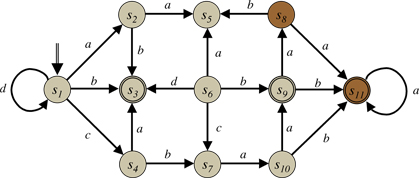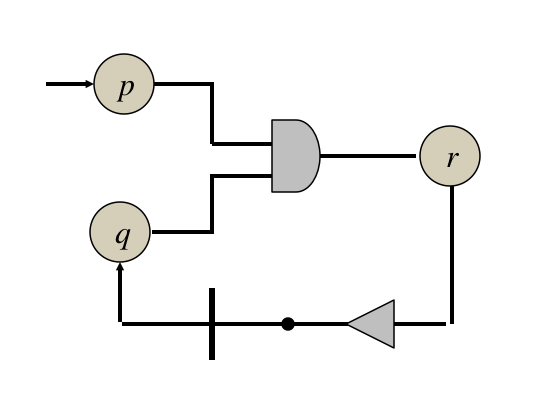C H A P T E R  13
 Propositional Nets

### 13.1 Introduction

In the introduction, we saw that it is possible to think of the dynamics of a game as a state graph. See, for example, the state graph in Figure 13.1. A game is characterized by a finite number of states, a finite number of players, each with a finite number of actions. At each point in time the game is in one of the possible states; players choose from their possible actions; and, as the players perform their chosen actions, the game changes from one state to another.Figure 13.1 - State Machine for a simple game

However, we rarely think of states as monolithic entities. In practice, we typically characterize states in terms of propositions that are true in those states. As actions are performed, some propositions become true and others become false. This suggests a conceptualization of games as propositional nets rather than state machines. A propositional net is a graph in which propositions and actions are nodes rather than states and where these nodes are interleaved with nodes representing logical connectives and transitions, as suggested by the example shown below.Figure 13.2 - Propositional Net for a simple game

One of the benefits of formalizing games as propositional nets is compactness. A set of n propositions corresponds to a set of 2n states (all different combinations of truth values for the n propositions). Thus, it is often possible to characterize the dynamics of games with graphs that are much smaller than the corresponding state machines. For example, the propnet in Figure 13.2, with just three propositions, corresponds to a state machine with eight states.

In this chapter, we start by formalizing propositional nets; we then show how to describe games in this way; and we talk about the properties of propositional nets. In the next chapter, we see how to use propositional nets in game playing. And in the chapters after that, we see how we can use propositional nets in recognizing structure in games and in discovering game-playing heuristics of various sorts.

### 13.2 Propositional Nets

A propositional net (propnet) is a directed bipartite hypergraph consisting of propositions alternating with connectives (inverters, and-gates, or-gates, and transitions). Propositions can be partitioned into three classes: input propositions (those with no inputs); base propositions (those with incoming arcs from transitions); and view propositions (those with incoming arcs from connectives other than transitions).

The propnet in Figure 13.3 is an example. In this case, there are six propositions (the nodes labelled a, b, p, q, r, and s); and there are four connectives (the and-gate on the upper left, the inverter on the upper right, the or-gate on the lower right, and the transition on the lower left). Nodes a and b are input propositions; node s is a base proposition; and nodes p, q, and r are view propositions.

Figure 13.3 - Sample propositional net

An input marking is a function from the input propositions of a propositional net to boolean values. A base marking is a function from the base propositions of a propositional net to boolean values. A view marking is a function from the view propositions of a propositional net to boolean values.

Given a propnet, an input marking and a base marking determine a unique view marking for that propnet. This is based on the types of gates leading into the view propositions. The output of an inverter is true if and only if its input is false. The output of an and-gate is true if and only all of its inputs are true. The output of an or-gate is true if an only if at least one of its inputs is true.

As an example, consider the propnet in Figure 13.3. Suppose we had an input marking that assigned a the value true and b the value false, and suppose we had a base marking that assigned s the value true. Then, the view marking for p would be true; the view marking for q would be false; and the view marking for r would be false. At this point, we have values for all of the view propositions in the propnet. See Figure 13.4. Here we have written 1 for true and 0 for false.

Figure 13.4 - One marking for a propositional net

Transitions are the basis for dynamics in a propnet. Consider a step in the operation of a propnet. Let us assume that there is an input marking and a base marking. From these, we can compute a view marking, as we have just seen. Importantly, this includes the inputs to the transitions of the propnet. On the next step, a new input marking is imposed from without. However, the new base marking is determined by the transitions. If the inputs to the transitions are true, then the outputs of the transitions are true on the next step. In effect, a transition as a mechanism for controlling the flow of information from one step to the next. It is a 1-step delay, a flip-flop in digital circuitry.

As an example, once again consider the propnet in Figure 13.3 with the marking illustrated in Figure 13.4. Now, let's move on to the next step. Suppose the input marking for the second step is the same as the first, i.e. a is true and b is false. What is s on this step? Since s is the output of a transition, its value on this step is the same as the value of that transition's input on the preceding step. In this case, the transition's input was false on the preceding step, and so s is false on this new step. As before, we can compute the view marking corresponding to the input marking and this new base marking. See Figure 13.5. In this case, since the second input to the and-gate is false, p is false and q is true and, therefore, r is true as well.

Figure 13.5 - Another marking

From this input marking and the new base marking, we can compute a new view marking. And we can then repeat. In this case, the the value of proposition s will go on alternating between true and false so long as input a is true and input b is false. If input a ever becomes false, it will stop alternating. However, the alternation will begin again as soon as it is set to true again.

### 13.3 Games As Propositional Nets

Propnets are an alternative to GDL for expressing the dynamics of games. With a few additional provisions, it is possible to convert any GDL game description into a propositional net with the same dynamics.

As an example of this, consider the simple game described below. There is just one role. There is just one base proposition, and there are two actions. The two actions are always legal. The player gets 100 points in any state in which s is true; otherwise, the player gets 0 points. The game ends if q ever becomes true. (Note that termination here is defined indirectly in terms of the action of the player. Properly, it should be defined entirely in terms of the state of the game and nothing else. This shortcoming can be fixed in various ways, but doing so would complicate the example.)

 ``` role(white) base(s) input(white,a) input(white,b) legal(white,a) legal(white,b) p :- does(white,a) & true(s) q :- ~p r :- q r :- does(white,b) next(s) :- r goal(white,100) :- true(s) goal(white,0) :- ~true(s) terminal :- q ```

Now, let's build a propnet for this game. The base propositions in the propnet consist of the propositions defined by the base relation in the game description (viz. s). The input propositions correspond to the actions defined by the input relation in the game description (viz. a and b).

We use the next relation to capture the dynamics of the game. Starting with the base and input propositions, we add links for each rule (using inverters for negations, and-gates for multiple conditions, and or-gates for multiple rules). In so doing, we augment the propnet with additional view propositions as necessary. The result is the propnet shown in Figure 13.3.

We model terminal in the propnet by adding a special node for termination. If necessary, we can extend the propnet to include new view propositions, as we do for next. In this case, terminal corresponds exactly to q, so we could just use q as our terminal node. However, for the sake of clarity, we can add a new node t and insert a connection from q to t. See Figure 13.6. Note that we use a one-input and-gate here. We could equally well use a two-input and-gate with both inputs supplied by q, but this is simpler. The behavior is the same.

Figure 13.6 - Propnet with terminal node

Rewards are handled analogously. We create a new node for each reward value, and we use the definitions for these values to extend the propnet further. In this case, goal(white,100) corresponds exactly to s, so we do not need to add a new node for goal(white,100). However, for clarity, in our example, we have added one node for each of the two goal values. See Figure 13.7.

Figure 13.7 - Propnet with goal nodes

Legality is the trickiest part. There are various ways of doing this. The simplest method conceptually is to add one legality propositions for each possible action and extend the propnet to say when these nodes are true. In this case, we add two new propositions la and lb, corresponding to actions a and b. In this case they are always true. To model this, we add a self-loop - a transition for each of these legality propositions - and we initialize to true. Because of the dynamics of transitions, these propositions will remain true indefinitely.

Figure 13.8 - Propnet with legality nodes

That's it. Once this is done, we have a propnet that reflects the game described in the given GDL. In the next chapter, we discuss how to use this propnet to play games.

### Exercises

Exercise 13.1: Consider the propnet shown in Figure 13.3. Assume a base marking that assigns s the value 0, and consider an input marking that assigns a the value 0 and b the value 1.

 (a) What is the marking of p on this step? (b) What is the marking of q on this step? (c) What is the marking of r on this step? (d) What is the marking of s on this step? (e) What is the marking of s on the next step?

Exercise 13.2: Consider the propnet shown in Figure 13.8. Assume a base marking that assigns s the value 1, and consider an input marking that assigns a the value 1 and b the value 0.

 (a) What is the player's reward in this state? (b) Is the state terminal?